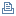Lotus Sandbox (1999-2007) <- domquery.info -> FeedBack & QuestionsArraySort

Sandbox sample submitted by Scott McIntosh.

An array sort using a fast shell sort algorthimCategory:Code Example
Audience:Application Developer
Product:Lotus Domino Document Manager (Domino.Doc)
QuickPlace
Notes/Domino
SmartSuite
Release:4 and higher
Platform:All Supported Platforms
Date Posted:12/04/2003
File Name:sort.zip
File Size:44,158 bytesClick here to download

Detailed Description:
Uses a shell sort algorithm to quickly sort an array. The code is in the Sort Test agent. The sub is ArraySort(). The code is shown below, but the agent also has code to demonstrate the routines use, test the routine, as well as compare it to a bubble sort. The speed difference is astounding.

============= Code =========================
``` '''''''''''''''''''''''''''''' ' ArraySort ' ' Sorts an array of values using a Shell Sort algorthim (ported from' a C language algorthim). ' ' Scott McIntosh ' ICF Consulting ' Rights are given to freely distribute or modify this code in any ' way that is useful. If you make improvements I would appreciate ' hearing about them. ' ' I can be contacted at smcintosh@icfconsulting.com ' ' Arguments: ' Boolean descending ' If passed "true" the array is sorted in decending order, otherwise ' it is sorted in ascending order. ' ' Should work for any data type that can be compared with the "<" and ' ">" operators, and can be modified easily to allow for the custom ' sorting of any object. ' Private Sub ArraySort( array As Variant, descending As Boolean) Dim aSpans(1 To 9) As Integer Dim nSpanCount As Integer Dim nSpanIncr As Integer Dim nLimit As Integer ' The number of items to sort Dim nSpan As Integer Dim KeyNum As Integer Dim SwapEm As Integer Dim SubArray(1 To 3) As Variant Dim Record1Keys As Variant Dim Record2Keys As Variant Dim doc As notesDocument Dim i As Integer Dim j As Integer Dim k As Integer Dim Temp As Variant Dim floor As Integer 'Define the spans used by the algorthim aSpans(1) = 9840 aSpans(2) = 3279 aSpans(3) = 1093 aSpans(4) = 364 aSpans(5) = 121 aSpans(6) = 40 aSpans(7) = 13 aSpans(8) = 4 aSpans(9) = 1 'The max spans possible nSpanCount = 9 'Which span to start on nSpanIncr = 1 floor = Lbound( array ) '' we use this a lot so store it nLimit = Ubound( array ) - floor If nLimit = 1 Then 'No need to sort a single element array Exit Sub End If ' Determine how many spans we'll have to make. ' this will depend on the number of elements to sort j = floor For k = nSpanCount To 1 Step -1 If aSpans(k) > nLimit Then ' The span exceeds the number of elements, exiting now retains the ' last span index, which will be the largest span less than the count Exit For End If j = k Next i = j For i = j To nSpanCount ' nSpanCount is the max number of spans we ' could make i is the first number from ' aSpans tha was less than the total ' number of documents nSpan = aSpans(i ) For j = nSpan To nLimit Temp = array(j) k = j - nSpan Do While k >= floor ' Compare and setermine if the values need swapped Dim doSwap As Boolean ' Determine which comparison to make based on the ' value of the argument passed in descending If descending Then doSwap = Temp > array(k) Else doSwap = Temp < array(k) End If ' If the comparison indicates they need swapped then ' do it If doSwap Then ' Swap 'em array(k + nSpan) = array(k) k = k - nSpan Else Exit Do End If Loop array( k + nSpan ) = Temp Next j Next i End Sub ```Document optionsPrint this pagePrint viewSearch

Sandbox viewsBy Application NameBy CategoryBy ProductBy AudienceBy DateBy SubmitterConferences

ResourcesdeveloperWorks: Lotus DownloadsalphaWorks Downloads